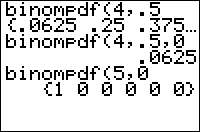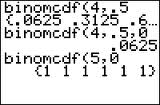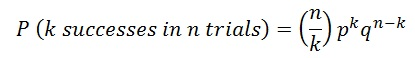# Difference Between BinomPDF and BinomCDF; Simple Steps to Enter Data

Share on

Probability and Statistics > Ti 83 for Statistics > Difference Between BinomPDF and BinomCDF

Contents:

## Difference Between BinomPDF and BinomCDF: Overview

Watch the video for an overview:

BinomPDF and BinomCDF are both functions to evaluate binomial distributions on a TI graphing calculator. Both will give you probabilities for binomial distributions. The main difference is that BinomCDF gives you cumulative probabilities.

## BinomPDF

BinomPDF is the probability that there will be X successes in n trials if there is a probability p of success for each trial. For example, if if n = 10, p = .5, and x = 3 the BinomPDF will return .117. That means there is a .117 (11.7%) chance that there will be 3 successes.

Use BinomPDF when you have questions that have:

• An exact number, like: all, half, none, or a specific number X.## BinomCDF

This function is exactly the same as BinomPDF except that instead of a specific number of successes (i.e. “3 trials”) this function gives you the probability there will be 0 to x successes in n trials. In other words, if you put X=3 it will five you the probability for 0,1,2 and 3 trials (all together). For example, if you input the same numbers as the BinomPDF example above (n = 10, p = .5, x = 3) you’ll get .172 (17.2%) with BinomCDF. This percentage is higher as it’s adding up all of the probabilities to X=3, much in the same way that you add probabilities in a cumulative frequency distribution table.

Use BinomCDF when you have questions with wording similar to:

• No more than, at most, does not exceed.
• Less than or fewer than.
• At least, more than, or more, no fewer than X, not less than X.
• Between two numbers (run BinomCDF twice).## BinomCDF TI 83 Calculator: OverviewThe TI 83 BinomPDF and TI 83 BinomCDF functions can help you solve binomial probability questions in seconds. Binomial probabilities involve two outcomes: Success or failure. For example, if you were tossing a coin to see how many heads you were going to get, if the coin landed on heads that would be a “success.” The difference between the two functions is that one (BinomPDF) is for a single number (for example, three tosses of a coin), while the other (BinomCDF) is a cumulative probability (for example, 0 to 3 tosses of a coin).

For single number probabilities (like two, or three, or a dozen, or something similar, you’ll want to use BinomPDF.

## BinomCDF TI 83 Calculator: Steps

Watch the video for the steps:

Example problem: Bob’s batting average is .321. If he steps up to the plate four times, find the probability that he gets up to three hits.

Step 1: Press 2nd VARS to get the “DISTR” option. Scroll down to “A:BinomCDF” and then press ENTER.

Step 2: Enter the number of trials. Bob bats four times, so the number of trials is 4. Press 4 followed by a comma.

Step 3: Enter the Probability of Success, P. Bob has a batting average of .321, so enter .321 followed by a comma.

Step 4: Enter the X value. We want to know what the probability is that Bob will get up to three hits, so enter 3, followed by a close parenthesis.

Step 5: Press ENTER for the result. Bob’s chances of getting up to three hits is .989.

Tip: Instead of scrolling down to “A:BinomCDF” you can press ALPHA then MATH.

That’s how to use BinomCDF TI 83 Calculator!

## Binomial Probability TI 83: BinomPDF.

This section tells you how to use the BinomPDF function to figure out an exact quantity (like three times, or twice, or a dozen occurrences). For cumulative probabilities (like “up to a certain number” or “between 5 and 10” or “under 6” or something similar, you’ll want to use BinomCDF.

Sample problem: Sue has a batting average of .270. If she is at bat three times, what is the probability that she gets exactly two hits?

Step 1: Press 2nd VARS to get the “DISTR” option. Press 0 for “binomPDF.”

Step 2: Enter the number of trials from the question. Sue bats three times, so the number of trials is 3. Press 3 and hit the , key.

Step 3: Enter the Probability of Success, P. Sue’s batting average is .270, so enter .270, then hit the , key.

Step 4: Enter the X value. We want to know Sue’s chances of getting exactly two hits, so enter 2 and then press ).

Step 5: Press for the result. Sue’s chances of getting exactly two hits is .159651.

Warning: BinomialPdf is an exact probability for one value of x. If you want to find a cumulative probability (for example, what are John’s chances of getting 0 or 1 hits?) you will need the use the BinomCDF function.

That’s how to find a Binomial Probability on the TI 83!

Check out our Youtube channel for more stats help and tips!

## References

Agresti A. (1990) Categorical Data Analysis. John Wiley and Sons, New York.
Everitt, B. S.; Skrondal, A. (2010), The Cambridge Dictionary of Statistics, Cambridge University Press.
Gonick, L. (1993). The Cartoon Guide to Statistics. HarperPerennial.
Vogt, W.P. (2005). Dictionary of Statistics & Methodology: A Nontechnical Guide for the Social Sciences. SAGE.

CITE THIS AS:
Stephanie Glen. "Difference Between BinomPDF and BinomCDF; Simple Steps to Enter Data" From StatisticsHowTo.com: Elementary Statistics for the rest of us! https://www.statisticshowto.com/difference-binompdf-binomcdf/
---------------------------------------------------------------------------Need help with a homework or test question? With Chegg Study, you can get step-by-step solutions to your questions from an expert in the field. Your first 30 minutes with a Chegg tutor is free!